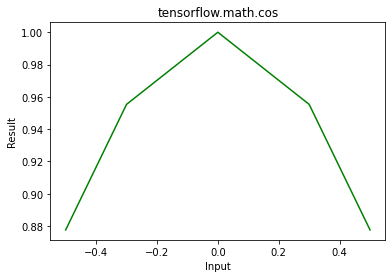Related Articles

# Python – tensorflow.math.cos()

• Last Updated : 17 Jun, 2021

TensorFlow is open-source python library designed by Google to develop Machine Learning models and deep learning  neural networks.
cos() is used to find element wise cos of x.

Syntax: tf.math.cos(x, name)

Parameters:

• x: It’s the input tensor. Allowed dtype for this tensor are bfloat16, half, float32, float64.
• name(optional): It’s defines the name for the operation.

Returns:  It returns a tensor of same dtype as x.

Example 1:

## Python3

 `# Importing the library``import` `tensorflow as tf` `# Initializing the input tensor``a ``=` `tf.constant([ ``-``.``5``, ``-``.``3``, ``0``, .``3``, .``5``], dtype ``=` `tf.float64)` `# Printing the input tensor``print``(``'Input: '``, a)` `# Calculating tangent``res ``=` `tf.math.cos(x ``=` `a)` `# Printing the result``print``(``'Result: '``, res)`

Output:

```Input:  tf.Tensor([-0.5 -0.3  0.   0.3  0.5], shape=(5, ), dtype=float64)
Result:  tf.Tensor([0.87758256 0.95533649 1.         0.95533649 0.87758256], shape=(5, ), dtype=float64)```

Example 2: Visualization

## Python3

 `# Importing the library``import` `tensorflow as tf``import` `matplotlib.pyplot as plt` `# Initializing the input tensor``a ``=` `tf.constant([``-``.``5``, ``-``.``3``, ``0``, .``3``, .``5``], dtype ``=` `tf.float64)` `# Calculating tangent``res ``=` `tf.math.cos(x ``=` `a)` `# Plotting the graph``plt.plot(a, res, color ``=``'green'``)``plt.title(``'tensorflow.math.cos'``)``plt.xlabel(``'Input'``)``plt.ylabel(``'Result'``)``plt.show()`

Output:Attention geek! Strengthen your foundations with the Python Programming Foundation Course and learn the basics.

To begin with, your interview preparations Enhance your Data Structures concepts with the Python DS Course. And to begin with your Machine Learning Journey, join the Machine Learning – Basic Level Course

My Personal Notes arrow_drop_up